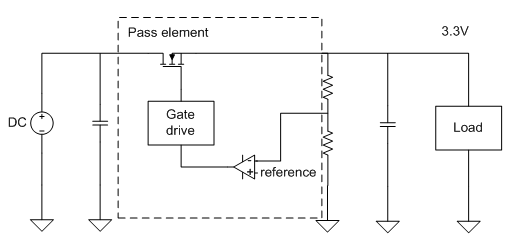# Watt’s wrong with my LDO?Choosing something as simple as a linear regulator is often a lesson in thermal dynamics. The designer usually realizes their error after the design is on the bench. Or worse, the design fails in the field because a regulator is running beyond its temperature rating. With all the new features and specifications of new linear regulators, it is easy to lose sight of the watts dissipated in the package.

Remember the linear regulator is basically a pass element and a controller. This element is a transistor that acts with the help of the control loop as a variable resistor which forms a voltage divider between the pass element and the load.Figure 1. Block diagram of the linear regulator.  Note the pass element that will form a voltage divider between it and the load, dissipating power.

People often overlook the fact that this is not a magical entity. Voltage is dropped across the pass element and it gets warmer. For example, if the circuit in figure 1 had a 100 mA constant load, it could be simplified and modeled for thermal purposes as shown in Figure 2. With an input of 5V, and output of 3.3V and an output of 100ma, the power dissipated by the pass element will be 170mW.

What if the input voltage was 24 volts? Now the power dissipated is (24-3.3) *100 mA = 2.07 Watts. This would be excessive heat in many tiny 150 ma regulators. Sure, we all know better. We all know V=I*R and P=I*V. But we let our minds trick us into thinking, “but it’s only 100 mA, or 50 ma, or so on.” Then the unforgiving laws of the universe sneak up and hit us in the back of the head in a very Gibbs-like fashion.

Figure 2. Simplified model of the linear regulator at steady state that shows where the power will be dissipated.

At the first order, this is a method I use to find a linear regulator. It is all about the package and, more importantly, the Watts in the package. If you calculate the power dissipation, you can very quickly move to choosing a package size.

1. Calculate power dissipation. Remember the linear regulator is just a variable resistor converting extra voltage drop to heat (Equation 1):

Pd= (Vi-VOUT)*IOUT                (Eq. 1)

2. Calculate the θjA required for the design’s desired maximum operating temperature. This is the junction’s thermal impedance specification to ambient temperature, based on package mounted on the PCB (degrees C/W). This isusually based on a typical maximum junction temperature of 150°C (note that some parts may have a lower max junction temperature, be sure to check the datasheet). The required θjA should be (Equation 2):

≤ (MaxJunctionTemp – MaxOperatingTemp)/Pd       (Eq. 2)

1. Filtering to devices in packages that allow for θjA less than the above calculation yields parts that meet this initial junction temperature requirement. Operating right at maximum junction temperature can affect reliability. It is always a good design practice to allow for some margin, depending on the board, airflow, environment and other nearby heat sources.
2. Once the list is narrowed based on thermal demands, it is much easier to hone in on other wish-list features, for example, fast transient response, power good, enable, low noise, and so on.
3. Always test your end result! A minute in the lab with a thermocouple can be more valuable than hours of calculations.

Simply calculate your thermals using this thermal calculator.

Thermal analysis can be examined deeper, but this first pass will help filter out parts that won’t work and guide you to parts that have a greater probability of working. The references provided go much deeper into this topic and show many more factors that can impact the thermal design.

References